## Clastic Sedimentary Rock Classification

Reading a Ternary Diagram and Creating Rock Names

A ternary diagram is a triangle with each of the three apexes representing a composition, such as sandstone, Shale, and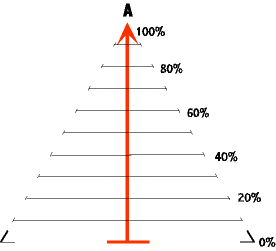Point A is at the top of the heavy vertical red line (arrow). Along this line is percent of A. A point plotted at the top of the vertical line nearest A indicates 100% A. A horizontal bar at the bottom of the line (farthest from A) represents 0% of A. Any other percentage can be indicated by a line located along the line between 0% and 100%, as shown by the numbers to the right of the diagram. The horizontal lines their percentage abundances are written along the right side of the triangle. By doing this the right side of the triangle becomes the scale for percent abundance of A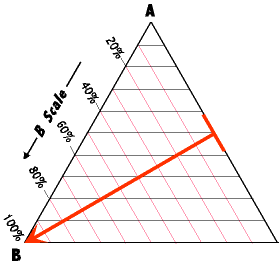Point B is at the lower left apex of the triangle. We construct a percent abundance scale for B by rotating the heavy red scale line 120 degrees counter clock wise so that it runs from the right side of the triangle to the lower left corner. The right side of the triangle now becomes the base line for the percent scale for B, and a series of red lines have been drawn parallel to the triangle's right side to mark the percentages. These lines are projected out to the left and bottom sides of the triangle, and the percent scale for B laid out along the left side.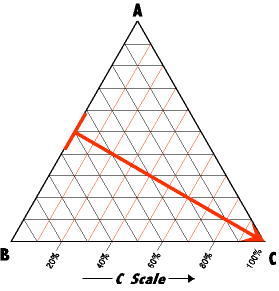Point C is at the lower right apex of the triangle.The percent abundance scale for C is constructed by rotating the heavy red scale line another 120 degrees so that it runs from the left side of the triangle to the lower right corner, and the percent scale lines and percent abundance numbers rotate with it. In the final ternary diagram the red vertical arrow is removed.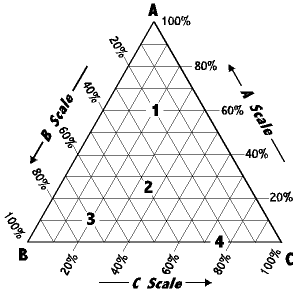The result is the ternary diagram to the left. Note that the heavy red lines are not included in this final triangle. Also observe that the ternary diagram is read counter clockwise.

~Naming Rocks From the Ternary Diagram~

Rock names must be logically and consistently derived so that one may easily and accurately go back and forth from diagram to rock name.Notice that the simple ideal model has three attractors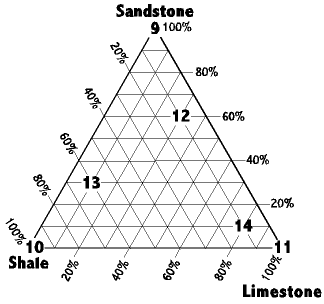(sandstone, Shale, and Limestone) and a ternary diagram has three apexes. Sandstone is labeled at the top, Shale in the lower left, and Limestone in the lower right.

Samples 9, 10, and 11 are at the apexes of the triangle and so each of these are pure end members, just as in the simple ideal model. However, any point that plots anywhere on one of the side lines, or within the triangle represents a rock composed of mixed end members.

The question here is, how would we name such rocks if we wanted to give them names? After all, saying something like "60% sand 30% Shale, 10% Limestone" for every rock would get tedious, and we would have to visualize the ternary diagram and plot the numbers to get a true sense of the rock.

Contributed by Lynn Fichter

Wednesday, October 15, 2014